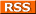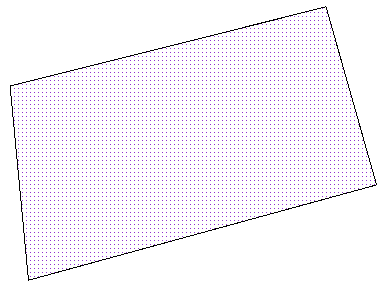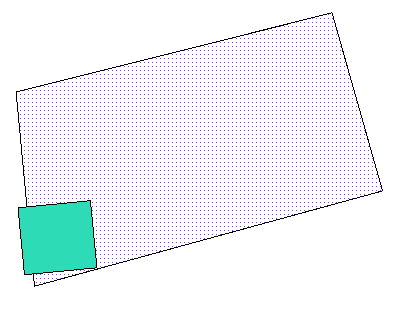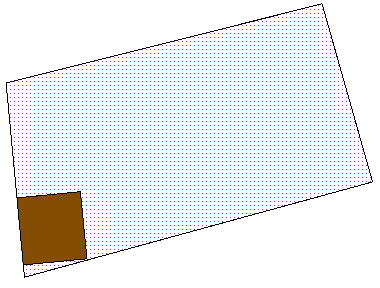Comments Rss
Intersects Intersection: PostGIS - ST_Intersects, ST_Intersection

# ST_Intersects, Intersects

ST_Intersects is a function that takes two geometries and returns true if any part of those geometries is shared between the 2. In PostGIS versions before 1.3 you would use the following syntax to utilize indexes ``` ```

``````SELECT a.somfield, b.somefield2
FROM a INNER JOIN b ON
(a.the_geom && b.the_geom AND intersects(a.the_geom, b.the_geom))
``````
``` ``` In versions from 1.3 forward, the && indexable operator is automatically included in the definition of ST_Intersects so you can simply write ``` SELECT a.somfield, b.somefield2 FROM a INNER JOIN b ON ST_Intersects(a.the_geom, b.the_geom) ```

## ST_Intersection, Intersection

The functions ST_Intersection and Intersection are compliments to ST_Intersects. What they return is that portion of geometry A and geometry B that is shared between the two geometries. If the two geometries do not intersect, then what is returned is an empty GEOMETRYCOLLECTION object.

NOTE: PostGIS versions 1.2.2 and up you should use ST_Intersection as Intersection is deprecated. The two functions are more or less equivalent though.

## Pictorial View of ST_Intersection

In this section we provide a graphical representation of what the Intersection looks like. We will use an example of a building and a parcel where the building does not completely sit inside the parcel.

``` ```

``````SELECT b.the_geom As bgeom, p.the_geom As pgeom,
ST_Intersection(b.the_geom, p.the_geom) As intersect_bp
FROM buildings b INNER JOIN parcels p ON ST_Intersection(b,p)
WHERE ST_Overlaps(b.the_geom, p.the_geom)
LIMIT 1;
``````
``` ```
 Parcel Geometry: pgeom:Building Geometry Overlayed on Top Of Parcel: bgeom: The building is in greenThe geometry returned by ST_Intersection(b.the_geom, p.the_geom) overlayed on top of Parcel: (intersection is in brown)Post Comments About Intersects Intersection: PostGIS - ST_Intersects, ST_Intersection

This Document is available under the GNU Free Documentation License 1.2 http://www.gnu.org/copyleft/fdl.html & for download at the BostonGIS site http://www.bostongis.com

Boston GIS      Copyright 2022      Paragon Corporation